Home | | Help | Contribute | Plug-ins | About | Contact | SearchFractal turtlesMarch, 2014

Note. It is best to read the original TeXmacs files with interactive Scheme sessions where one may experiment with the code. It can be found in the project's source code, inside the directory web/miguel.

Here we build upon what we previously did in “Turtle schemes”. In order to quickly load it a good method is to use Developer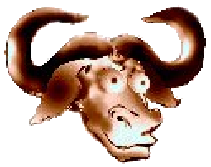Export sessions (your cursor will have to be inside one session to be enable this action) to save the contents of all Scheme sessions to a Scheme file, then load it from this file using (load "path/to/file.scm"). From now on, we assume all the functions defined in “Turtle schemes” are available.

For our first example we start with a simple case: we paint dots at each of the vertices of an equilateral triangle.

 Scheme] (define (three-dots sz) (let ((x _posx) (y _posy)) (map (lambda (ang) (go (list x y)) (look ang) (forward sz) (fill (circle 1) "black")) '(90 -30 210))))

 Scheme] (draw (three-dots 4))

It is obvious that one could paint anything at the vertices. For instance an ugly gnu (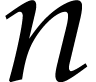) or the figure resulting of a recursion step as we next do. First we slightly generalize the previous code to draw any three things (this in turn may be easily generalized to an arbitrary regular polygon). Then we do the recursion in the function pinski: the argument fig is the figure which is drawn on each vertex of the triangle, count the number of iterations and sz the distance between figures. Play with the parameters, but before increasing the number of iterations save your document! An increment of one unit dramatically increases the number of dots.

 Scheme] (define (three-things thing sz) (let ((x _posx) (y _posy)) (map (lambda (ang) (go (list x y)) (look ang) (forward sz) (thing)) '(90 -30 210))))

 Scheme] (define (pinski fig count sz) (if (> count 1) (three-things (lambda () (pinski fig (- count 1) (* sz 0.5))) sz) (three-things fig sz)))

 Scheme] (draw (pinski (lambda () (fill (circle 0.2) "black")) 5 25))

Yes, that was (or should've been) the Sierpinsky triangle. As promised, we now generalize the previous code to a general regular polygon with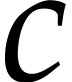vertices. We want to place these polygons recursively at the vertices of the previous polygon and for this we need a little computation for the scaling factor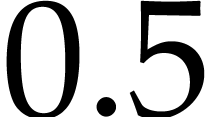(the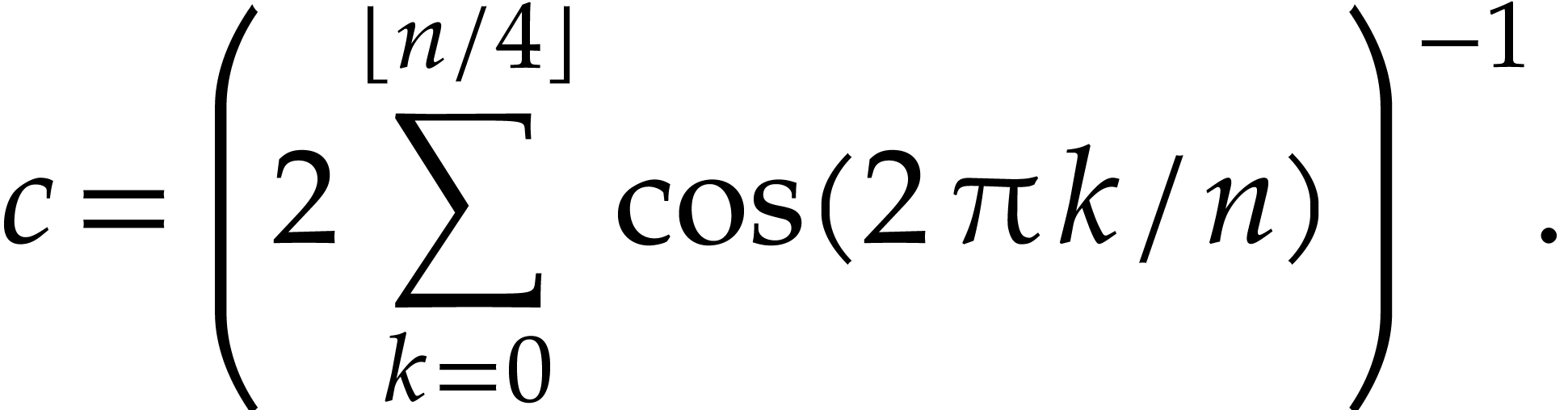that we silently introduced in pinsky). If you feel lazy just trust this formula and its implementation in n-factor:We also implement in a straightforward way the generalizations topolygons.

 Scheme] (define (n-factor n) (with a_k (lambda (k) (cos (/ (* 2 pi k) n))) (/ 1 (* 2 (apply + (map a_k (.. 0 (+ 1 (floor (/ n 4))))))))))

 Scheme] (define (n-angles n) (with ang (/ 360 n) (map (lambda (x) (floor (+ (- 90 ang) x))) (map (lambda (x) (* x ang)) (.. 1 (+ 1 n))))))

 Scheme] (define (n-things n thing sz) (let ((x _posx) (y _posy)) (map (lambda (ang) (go (list x y)) (look ang) (forward sz) (thing)) (n-angles n))))

 Scheme] (define (n-pinski n fig cnt sz) (with nextfig (lambda ()(n-pinski n fig (- cnt 1) (* sz (n-factor n)))) (if (> cnt 1) (n-things n nextfig sz) (n-things n fig sz))))

 Scheme] (draw (n-pinski 7 (lambda () (fill (circle 0.2) "black")) 4 30))

Just one more thing: you might want your output inline, but our previous plot will not work with complex drawings because they have to be simplified. Here's (yet another) quick hack:

 Scheme] (define (plot* . l) ; Remember the drawing contract: ; Drawing functions (such as turn) with no graphics output return '() (cond ((nlist? l) '(graphics "" "")) ((== l '()) (noop)) ((list? (car l)) ‘(graphics "" ,@(car l))) (else ‘(graphics "" ,@l))))

 Scheme] (define (plot l) (stree->tree (plot* (simplify l 0))))

 Scheme] (plot (n-pinski 5 (lambda () (fill (circle 0.2) "black")) 5 30))

Now try your own figures. Have fun!

Bonus: try redefining logo-canvas-extra to add a menu to pick the number of iterations and redraw.

This webpage is part of GNU TeXmacs and the larger GNU project. Verbatim copying and distribution of it is permitted in any medium, provided this notice is preserved. For more information or questions, please contact Joris van der Hoeven.

Free Software Foundation, Inc., 51 Franklin Street, Fifth Floor, Boston, MA 02111, USA